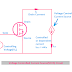## Definition/Concept

Voltage Controlled Current Source is that where the current is dependent or controlled by the changing of voltage elsewhere in the circuit. In short, it is known as VCCS. For dependent sources, sometimes voltage is controlled by current, and sometimes current is controlled by voltage. According to those, there are four types of dependent sources. Anyway, the basic concept of the VCCS is, that voltage controls the output current. So the output current Iout is proportional to the controlled input voltage Vin.

The voltage-controlled current output is determined by the equation, IOUT = αVIN

Here, IOUT  = Output Current
VIN = Input Voltage
α(alpha) = Multyplying Constant, sometimes it is known as transconductance of the current source.

Remember that the unit of  α(alpha) is mho because, α = IOUT/VIN

The unit comes from ampere/volt.

## Voltage Controlled Current Source(VCCS) Circuit Diagram

Here, you can see a very simple and basic circuit diagram of VCCS. We just take a field-effect transistor where a controlling voltage source is shown on the gate terminal side and the controlled current source is shown in the source and drain terminal.

Here, you can see the voltage across the gate and source terminal of the field-effect transistor is considered as a controlling voltage. And the Current flowing through the source to the drain terminal is considered as a dependent current or controlled current. we know that the changes in voltage to the gate terminal of the field-effect transistor can change the drain current. So if there is a current source connected across the drain and source terminal it will be a controlled current source that is controlled by the gate voltage.

## VCCS Symbol

Here, you can see the symbol of voltage-controlled current source. The left section shows the controlled input voltage whereas the right section shows the dependent current source that is controlled by the voltage.

## VCCS Example

A practical example of VCCS is Field Effect Transistor(FET). Yes, because here the gate voltage controlled the drain current. You will see the applications of Voltage control current source in digital to analog converter circuits.

What is VCCS(Voltage Controlled Current Source)? ExamplesReviewed by Author on June 18, 2022 Rating: 5